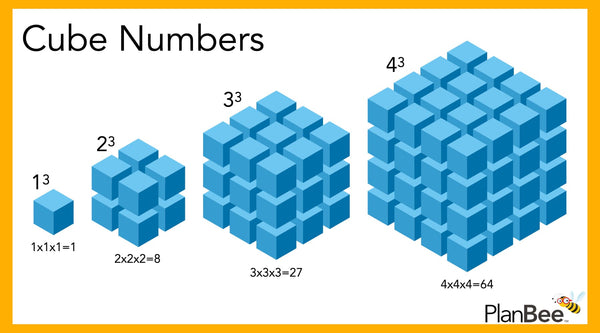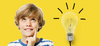# Cube Numbers

## What are cube numbers?

A cube number is the product of a number multiplied by itself twice.

In maths, the word ‘product’ means the result of one or more multiplications. For example, in the number sentence, axb=c (a multiplied by b equals c), c is the product of the equation. In the number sentence, axaxa=b (a multiplied by a multiplied by a equals b), b is the product of the equation.

Cube numbers include 1, 8, 27, 64 and 125, because

1x1x1=1

2x2x2=8

3x3x3=27

4x4x4=64

5x5x5=125

… and so on!

## Why are cube numbers called cube numbers?

Cube numbers are called cube numbers (or cubed numbers) because they form the area of a cube. A cube is a 3D shape with an equal number of units in length, width, and height so they make a cube.A diagram showing 13 to 43

## Here is a list of all the cube numbers to 1000:

 Number Cube Cube roots 1 1 1x1x1 2 8 2x2x2 3 27 3x3x3 4 64 4x4x4 5 125 5x5x5 6 216 6x6x6 7 343 7x7x7 8 512 8x8x8 9 729 9x9x9 10 1000 10x10x10

Try covering one of the columns, can you fill in the missing numbers?

Can you work out the cube numbers up to 8000?

## Is there a quick way to write a number cubed?

Yes! Numbers that are going to be cubed are often written with a little 3 after them.

13 means 1x1x1 so 12 = 1

23 means 2x2x2 so 23 = 8

33 means 3x3x3 so 33 = 27

43 means 4x4x4 so 43 = 64

53 means 5x5x5 so 53 = 125

## When are cube numbers taught in the English National Curriculum?

In the English National Curriculum, cube numbers are introduced to children in Year 5. They build on their knowledge of multiplication and division by learning the notations for squared and cubed. They then use this knowledge to solve problems.

You can find all our Maths schemes of work featuring cube numbers by using our objective checker.

Teach your Year 5’s about Squares, Cubes, and Factors. During this five-lesson series, your class will learn how to identify and use squared and cubed numbers, as well as how to find factor pairs. They will find factor pairs using divisibility tests to identify factors of larger numbers.

Revisit the children’s understanding of square and cube numbers in the context of area and volume with our Primes, Squares, and Cubes series of lessons. Then move on to explore the definition of prime and composite numbers and their properties.

If you are looking for other primary school maths lessons, make sure to have a look at our collection of maths packs, which are designed to give you full coverage of the national curriculum.

If you are looking for some free resources to support your maths teaching, look no further! We have stacks of printable free maths resources and an informative square number blog ready and waiting for you

### Related Posts##### Anglo-Saxon KS2 Facts
Where did the Anglo-Saxons come from? What did the Anglo-Saxons eat? What did they wear? Find out some fascinating facts##### How to make children more energy aware
With the current energy crisis in full swing, and costs rising at an alarming rate for schools who are already strugglin